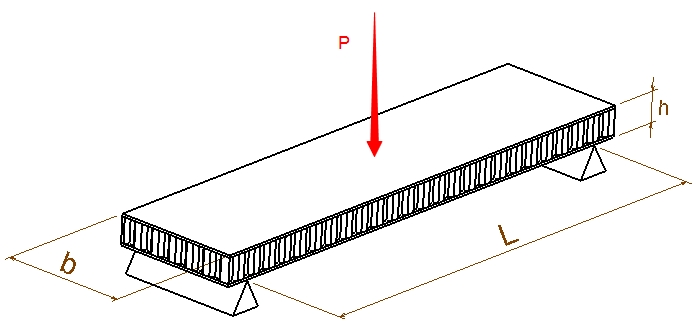Composite Sandwich Panel Stress Calculator
Hits: 13415
This is the Composite Sandwich Panel (Simple Supported) Bending Stress, Core Stress, Ply(skin) Stress, Stiffness & Deflection Calculator - Simply enter the values to calculate the design parameters of the composite sandwich panel.P (lbs) = tc (inch) = tf (inch) = Ef (psi) = b (inch)= L (inch)= Gc (psi) = P is the applied load on the panel.tc is the composite panel core thickness.tf is the composite panel skin(ply) thickness.Ef is the elasticity modules of the skin.b is the width of the panel.L is the distance between supports.Gc is the shear modules of the core. If you don't know the shear modules of the core leave it blank.